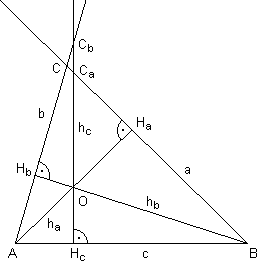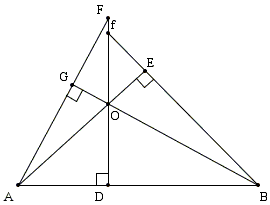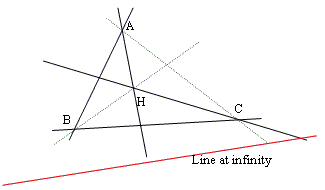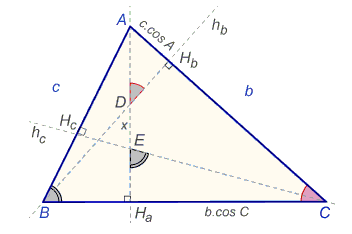# Existence of the Orthocenter

In a triangle, an altitude is a segment of the line through a vertex perpendicular to the opposite side. An altitude is the portion of the line between the vertex and the foot of the perpendicular. Using the standard notations, in $\Delta ABC$, there are three altitudes: $AH_{a},$ $BH_{b},$ $CH_{c}.$ The altitudes have several very interesting properties; below we are concerned with just one: the three lines meet at a point - the orthocenter of the triangle (denoted $H.)$ For an obtuse triangle (having one angle exceeding 90°), the orthocenter lies outside the triangle, and the segments $AH_{a},$ $BH_{b},$ $CH_{c}$ do not meet. However, their extended lines do, so even in this case, it is common to assert that the altitudes are concurrent, that is pass through a point.

20 February 2015, Created with GeoGebra

This is a matter of real wonderment that the fact of the concurrency of altitudes is not mentioned in either Euclid's Elements or subsequent writings of the Greek scholars. The timing of the first proof is still an open question; it is believed, though, that even the great Gauss saw it necessary to prove the fact. The earliest known proof was given by William Chapple (1718--1781). It is listed below, but appears on a separate page along with historical remarks.

Let's observe that, if $H$ is the orthocenter of $\Delta ABC$, then $A$ is the orthocenter of $\Delta BCH,$ while $B$ and $C$ are the orthocenters of triangles $ACH$ and $ABH,$ respectively.

I have collected several proofs of the concurrency of the altitudes, but of course the altitudes have plenty of other properties not mentioned below. For example, due to the mirror property the orthic triangle solves Fagnano's Problem. The foot of an altitude also has interesting properties.1. Altitudes as Cevians

This is Corollary 3 of Ceva's theorem.

2. Orthocenter as Circumcenter

The orthocenter of $\Delta ABC$ coincides with the circumcenter of $\Delta A'B'C'$ whose sides are parallel to those of $\Delta ABC$ and pass through the vertices of the latter.

3. Orthocenter as the Isogonal Conjugate of the Circumcenter

Besides being the bisector of angle $A,$ $l_{a}$ also bisects the angle formed by $h_{a}$ and the diameter of the circumscribed circle that contains $A.$ It follows that that diameter and $h_{a}$ are isogonal images of each other. The same is true for the vertices $B$ and $C.$ Therefore, $H$ is the isogonal conjugate of the circumcenter O.

4. Orthocenter as Incenter

In $\Delta ABC$, $\Delta H_{a}H_{b}H_{c}$ is known as the orthic triangle. It has an interesting property that its angle bisectors serve in fact as altitudes of $\Delta ABC$. Thus, the fact that, in a triangle, angle bisectors are concurrent, implies the fact that altitudes in a triangle are also concurrent.In the proof I shall repeatedly use Euclid's Proposition III.21 about inscribed angles and its reverse. Angles $BH_{c}C,$ $AH_{a}B,$ $AH_{a}C,$ $BH_{b}C$ are all right. Therefore, we get three quadrilaterals inscribable in a circle: $BH_{c}HH_{a},$ $BH_{c}H_{b}C,$ and $CH_{b}HH_{a}.$ In each, there is a pair of equal angles. Respectively: $\angle H_{c}BH=\angle H_{c}H_{a}H,$ $\angle H_{c}BH_{b}=\angle H_{c}CH_{b},$ and $\angle H_{b}CH=\angle H_{b}H_{a}H.$ It remains only to note that, naturally, $\angle H_{c}BH= \angle H_{c}BH_{b}$ and $\angle H_{b}CH=\angle H_{b}CH_{c}.$ Finally, $\angle H_{c}H_{a}H=\angle H_{b}H_{a}H,$ which proves that $H_{a}H$ is an angle bisector in the orthic triangle. The other two angles are treated similarly.

5. Via the Euler Line

The argument that shows that three points - the circumcenter $O$, the centroid $M,$ and the orthocenter $H$ - lie on the same line is reversible.

Indeed, in $\Delta ABC$ consider the centroid $M$ and the circumcenter $O.$ If they coincide, then so are the corresponding medians and the perpendicular bisectors. In other words, the medians are perpendicular to the sides and, therefore, coincide with the altitudes. The altitudes then intersect at the centroid of the triangle (which is obviously equilateral in this case.)

Assume that the points $O$ and $M$ are distinct. They define a unique line on which we'll consider a point, denoted as $H,$ such that $MH=2\cdot OM$ with $M$ lying between $O$ and $H.$ Since also $AM = 2\cdot MM_{a},$ $\Delta AHM$ is similar to $\Delta M_{a}OM.$ Elements VI.2 implies that lines $OM_{a}$ and $AH$ are parallel. But the former is perpendicular to $BC$ and, therefore, so is the latter. Similarly, $BH \perp AC$ and $CH \perp AB.$

6. Complex Variables

A proof in the circular coordinates leads directly to the Euler line and a nice theorem by J. L. Coolidge

7. Complex Variables II

Two short proofs of which the second is the clearest proof I ever came across.

8. Vector Algebra I

Given $\Delta ABC$, select any point O as the origin and consider vectors $OA,$ $OB$, and $OC$ that start at O and end at the vertices of the triangle. Introduce "side" vectors: $AB=OB-OA,$ $BC=OC-OB,$ and $AC=OC-OA.$ In a similar manner, other vectors will be used that lie along straight lines associated with the triangle. Assume $H$ is the point of intersection of $AH_{a}$ and $BH_{b}.$ Then $AH\perp; BC$ and $BH \perp AC.$ The scalar product of orthogonal vectors is $0.$ We thus have two equations

$(OH - OA)\cdot (OC - OB)$ = 0 and $(OH - OB)\cdot (OC - OA) = 0.$

Subtract the first equation from the second, multiply out and simplify:

$OH\cdot OB + OA\cdot OC - OB\cdot OC - OH\cdot OA = (OH - OC)\cdot (OB - OA) = CH\cdot AB = 0$

Therefore $CH\perp AB.$ Thus the third altitude $CH_{c}$ passes through the point of intersection of the first two.

9. Vector Algebra II

Let now $O$ be the circumcenter of $\Delta ABC$. Define $H$ via $OH = OA + OB + OC.$ We are going to show that $H$ lies on each of the altitudes of $\Delta ABC$. For example,

\begin{align} AH\cdot BC &= (AO + OH)\cdot (BO + OC)\\ &= (-OA + OA + OB + OC)\cdot (OC - OB)\\ &= (OB + OC)\cdot (OC - OB)\\ &= OC\cdot OC - OB\cdot OB\\ &= OC^{2} - OB^{2}\\ &= 0, \end{align}

because O is the circumcenter of $\Delta ABC$.

10. Vector Algebra III

This is very much like the first proof by Vector Algebra, but we start with an identity that is valuable in its on right. The identity is attributed to L. Euler.

$AB\cdot CD + AC\cdot DB + AD\cdot BC = 0.$

Chosing an arbitrary point $O$ as the origin, for a generic point $X$ let $x$ denote vector OX.$then the above identity is equivalent to$(b-a)\cdot (d-c) + (c-a)\cdot (b-d) + (d-a)\cdot (c-b) = 0.$This can be shown purely algebraically:$\begin{align} (b-a)\cdot (d-c) &= b\cdot d-a\cdot d-b\cdot c+a\cdot c,\\ (c-a)\cdot (b-d) &= c\cdot b-a\cdot b-c\cdot d+a\cdot d,\\ (d-a)\cdot (c-b) &= d\cdot c-a\cdot c-d\cdot b+a\cdot b. \end{align}$Adding the three up cancels all the terms on the right, making the sum on the left$0.$Now, let's use that identity with$D=H$being the intersection of the altitudes from, say$A$and$B.$This means that$AC\cdot HB=0$and$AH\cdot BC=0,$such that also$AB\cdot CH=0.$11. Elementary Geometry, Inscribed Angles Thanks to Bianco for this proof. See also Altshiller-Court's College Geometry, p. 94. Let$H$be the point of intersection of two altitudes$BH_{b}$and$CH_{c}.$We are going to prove that the line$AH$is perpendicular to$BC.$The quadrilateral$CH_{b}H_{c}B$is cyclic. Indeed, since the angles at$H_{b}$and$H_{c}$are right, the quadrilateral is inscribed in the circle with diameter on$BC.$From here,$\angle BCH_{c}=\angle BH_{b}H_{c}.$On the hand, the quadrilateral$AH_{b}HH_{c}$is also cyclic, as the circle with diameter$AH$passes through all four points. Therefore,$\angle HH_{b}H_{c} = \angle HAH_{c}.$Combining the two equalities, we get$\angle BCH_{c}=\angle HAH_{c}.$Extend$AH$beyond$H.$Let$G$be the point of intersection of$AH$and$BC.$In triangles$CHG$and$AHH_{c},\angle GCH = \angle HAH_{c}$and also$\angle CHG = \angle AHH_{c}.$The triangles are therefore similar. Which implies that$\angle HGC = \angle HH_{c}A = 90^{\circ}$making$CG$the third altitude. 12. Plain Analytic Geometry (Vladimir Zajic.) Assume a triangle$ABC$in a carthesian coordinate system. Assume that no side is parallel to any of the$2$coordinate axes$(x, y).$If yes, we can always rotate the coordinate system by an arbitrary angle different from all triangle interior angles. Since the coordinate axes$x$,$y$are perpendicular to each other and since each altitude is perpendicular to one side, it follows that no altitude is parallel to any coordinate axis either. Let the coordinates of the$3$vertices be:$\begin{align} A = (x_{a}, y_{a}),\\ B = (x_{b}, y_{b}),\\ C = (x_{c}, y_{c}). \end{align}$Equations of the$3$side lines are$\begin{align} c &= AB: y - y_{a} = {(y_{a} - y_{b})\cdot x + x_{a}\cdot y_{b} - x_{b}\cdot y_{a}} / (x_{a} - x_{b})\\ a &= BC: y - y_{b} = {(y_{b} - y_{c})\cdot x + x_{b}\cdot y_{c} - x_{c}\cdot y_{b}} / (x_{b} - x_{c})\\ b &= CA: y - y_{c} = {(y_{c} - y_{a})\cdot x + x_{c}\cdot y_{a} - x_{a}\cdot y_{c}} / (x_{c} - x_{a}). \end{align}$We have to calculate only$1$equation, the other$2$are given by cyclic permutation of indices$A,B,C.$### Lemma Two lines (none parralel to any coordinate axis) are perpendicular to each other if and only if the product of their tangents is equal to -1 (minus one). Equations of the 3 altitudes CH_{c}, BH_{c}, AH_{a} are obtained by using the tangents of the side lines, the lemma, and the fact that they pass through the corresponding vertex. Again, we have to set up only 1 equation, the other 2 are given by the cyclic permutation of A, B, C.$\begin{align} CH_{c}: y - y_{c} &= {-(x_{a} - x_{b})\cdot x + (x_{a} - x_{b})\cdot x_{c}} / (y_{a} - y_{b})\\ AH_{a}: y - y_{a} &= {-(x_{b} - x_{c})\cdot x + (x_{b} - x_{c})\cdot x_{a}} / (y_{b} - y_{c})\\ BH_{b}: y - y_{b} &= {-(x_{c} - x_{a})\cdot x + (x_{c} - x_{a})\cdot x_{b}} / (y_{c} - y_{a}). \end{align}$To find the coordinates of the intersect (the orthocenter), take any two altitude equations and solve for$x$and$y.$For example,$CH_{c} \cap BH_{b}:\begin{align} x_O &= (x_{a}\cdot x_{b}\cdot (y_{a} - y_{b}) + x_{b}\cdot x_{c}\cdot (y_{b} - y_{c})\\ &+ x_{c}\cdot x_{a}\cdot (y_{c} - y_{a}) - (y_{a} - y_{b})\cdot (y_{b} - y_{c})\cdot (y_{c} - y_{a}))\\ & / (x_{c}\cdot y_{b} - x_{b}\cdot y_{c} + x_{a}\cdot y_{c} - x_{c}\cdot y_{a} + x_{b}\cdot y_{a} - x_{a}\cdot y_{b}) \end{align}\begin{align} y_O &= (y_{a}\cdot y_{b}\cdot (x_{a} - x_{b}) + y_{b}\cdot y_{c}\cdot (x_{b} - x_{c})\\ &+ y_{c}\cdot y_{a}\cdot (x_{c} - x_{a}) - (x_{a} - x_{b})\cdot (x_{b} - x_{c})\cdot (x_{c} - x_{a})) \\ & / (y_{c}\cdot x_{b} - y_{b}\cdot x_{c} + y_{a}\cdot x_{c} - y_{c}\cdot x_{a} + y_{b}\cdot x_{a} - y_{a}\cdot x_{b}) \end{align}$Since the solution is invariant with respect to the cyclic permutation of A, B, C, it follows that the same coordinates xO and yO are solution of any two altitude coordinates and the 3 altitudes indeed intersect in a single point. This could be also verified by a direct solution of all altitude equation pairs. 13. Plane Geometry (Vladimir Zajic.) Assume a$\Delta ABC$, the side$c = AB$is horizontal, the vertex$C$is above. Extend the sides$a = BC$and$b = CA$upwards beyond the vertex$C.$Construct the altitudes$h_{a}$and$h_{b}$by dropping normals from the vertices$A$and$B$to the opposite sides$a = BC$and$b = CA,$respectively. Denote$O$the intersection of these two altitudes. Denote$H_{a}$and$H_{b}$the feet of the altitudes$h_{a}$and$h_{b},$respectively (i.e., their intersections with the corresponding triangle sides). Construct the altitude$h_{c}$of the$\Delta ABO$by dropping a normal from the vertex$O$to the side$c = AB.$Denote$h_{c}$the foot of this altitude. Extend the altitude$h_{c}$of the$\Delta ABO$upwards until it intersects both the (extended) lines$a = BC$and$b = CA.$Suppose that these intersections might be different from each other (see the attached drawing). Denote the intersections$C_{a}$and$C_{b},$respectively. Then either$OC_{a} \lt OC_{b}$or$OC_{a} \gt OC_{b}.$Note that the following triangle pairs are similar (because both triangles in each pair have the same angles at the vertex$O$and each triangle has a right angle):$\Delta AOH_{b}$and$\Delta BOH_{a}\Delta AOH_{c}$and$\Delta C_{a}OH_{a}\Delta BOH_{c}$and$\Delta C_{b}OH_{b}$Consequently$OA/OH_{b} = OB/OH_{a}OA/OH_{c} = OC_{a}/OH_{a}OB/OH_{c} = OC_{b}/OH_{b}$Eliminating$OA$and$OH_{a}$by dividing the left sides and the right sides of the first two equations we get$OH_{c}/OH_{b} = OB/OC_{a}.$Multiplying the left sides and the right sides of the result and of the third equation eliminates almost everything else:$OB/OH_{b} = OC_{b}/OH_{b}\cdot OB/OC_{a}OC_{b}/OC_{a} = 1$The line segments$OC_{b}$and$OC_{a}$are equal. In other words, the lines$a$and$b$intersect on the normal$h_{c}$to the line$c$dropped from the point$O$and the points$C,C_{b},$and$C_{a}$are identical. Q.E.D. 14. Altitudes As Radical Axes(Vladimir Zajic.) Form three circles$C_{a},C_{b},$and$C_{c}$on the sides$BC,AC,$and$AB$of$\Delta ABC$as diameters. Circles$C_{a}$and$C_{b}$meet at$C$and one other point. This point lies on$AB$and is in fact the foot$h_{c}$of the altitude$CH_{c}.$Indeed, let$K$be the point of intersection (other than$B$) of$C_{a}$with$AB.$Then$\angle CKB = 90^{\circ},$so that$CK$is perpendicular to$AB.$Therefore$K = H_{c}.$Similarly, circle$C_{b}$meets$AB$at$h_{c}$(and, of course,$A.)$We conclude that two circles$C_{a}$and$C_{b}$that obviously meet at$C,$also meet at$H_{c}.CH_{c}$is therefore the radical axis of the two circles. Turning to other sides and pairs of circles, we see that the altitudes of$\Delta ABC$serve as radical axes of the circles$C_{a},C_{b},$and$C_{c}$taken in pairs. As we know, the pairwise radical axes of three circles concur in a point, and so do the three altitudes of a triangle. (A more general statement appears as Theorem 184 in A Treatise On the Circle and the Sphere by J. L. Coolidge: The orthocenter of a triangle is the radical center of any three circles each of which has a diameter whose extremities are a vertex and a point on the opposite side line, but no two passing through the same vertex. The proof is practically the same.) 15. Via Carnot's Theorem The identity (1)$AC'^{2} - BC'^{2} + BA'^{2} - CA'^{2} + CB'^{2} - AB'^{2} = 0.$in Carnot's theorem is easily verified when$AA',BB',CC'$are the altitudes of$\Delta ABC$. 16. A Proof from 1749 A former correspondent sent me a surprise note (Jan 20, 2010): Here is William Chapple's demonstration that the altitudes of a triangle are coincident. It comes from the Miscellanea Curiosa Mathematica, Number IX, edited by Francis Holliday and published by Cave - I understand that this publication was launched in 1745, with two issues a year, which would place the publication date in 1749, the year of publication of a collected edition ... William Chapple's demonstration refers to the following diagram:I placed the proof itself on a separate page along with a commentary by Thomas Stephens Davies in The Philosophical Magazine in 1850. 17. And a More Recent Proof This proof is due to V. Nikolin, an elementary school teacher from Serbia, and is based on the following diagram:The short proof is available on a separate page. 18. Lightened Analytic Proof This and the next 3 proofs are due to Michel Cabart (Feb 1, 2011).Let's take as$x$-axis the side$BC$and as$y$-axis the altitude$AH,$so that the origin is$H.$The coordinates are$A(0, a),B(b, 0)$and$C(c, 0).$We want to show that intersections$L$and$K$have same ordinate. Slope of$AB$is$-a/b$hence slope of$CC'$is$b/a$and equation of$CC'$is$y = (b/a)\cdot (x - c) = (b/a)x -(bc/a).$Thus ordinate of$K$is$-bc/a.$This is symmetric in$b$and$c,$so we are sure to find the same result for$L.$19. Short Geometric Proof With a reference to the diagram in the previous proof, triangles$AHB$and$CHL$are similar (rectangle triangles with$\angle HAB = \angle HCL),$thus$HB\cdot HC = HA\cdot HL.$By symmetry (or considering triangles$AHC$and$BHK)$we get$HB\cdot HC = HA\cdot HK.$Hence$HK = HL$and$K = L.$20. Projective Proof (Chasles) Let's consider the quadrilater HABC in this order,$H$being any point.From Desargues' theorem, the line at infinity cuts$AB$and$CH,AC$and$BH,BC$and$AH$in three pairs of points in involution:$C' - H1,B' - H2,A' - H3.$If two pairs of lines are perpendicular, the double points of the involution are the cyclic points. Then the third pair of lines is also perpendicular. 21. A Trigonometric Proof by Dušan Vallo A proof illsutrated by the following diagram has been placed on a separate page.22. Concurrence of the Altitudes As Seen from 3D Stuart Anderson came up with a proof that benefits from embedding the triangle into$3D.\$A dynamic illustration is also available.

(By the way, You may be surprised to learn that altitudes have ears, foot, stem, and root.)

### References

1. N. Altshiller-Court, College Geometry, Dover, 1980
2. J. L. Coolidge, A Treatise On the Circle and the Sphere, AMS - Chelsea Publishing, 1971
3. H. S. M. Coxeter, S. L. Greitzer, Geometry Revisited, MAA, 1967
4. H. S. M. Coxeter, Introduction to Geometry, John Wiley & Sons, 1961
5. D. Pedoe, Geometry: A Comprehensive Course, Dover, 1970
6. V. V. Prasolov, Essays on Numbers and Figures, AMS, 2000•